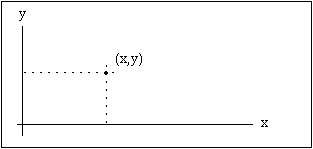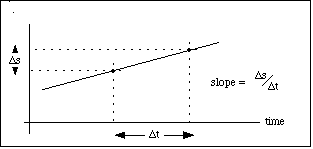Hands-On-Physics

The Great Bungee Jump Concepts: MATHEMATICAL MODELS

One of the main strengths of physics is its use of mathematical modelling. Here are some formulas which may be useful for modelling in the Great Bungee Jump unit.

Force

The relationship between force and motion is central to the model of nature which we call Physics.

• F = ma

{F = force in newtons, m=mass in kg., a=acceleration in meters/second/second}

Freefall

• s = vi*t + 1/2 g*t^2
{s = distance fallen, vi = initial velocity, t = time in seconds
g = acceleration of gravity = 9.81 m/s/s }

• If vi*t = 0, then s = 1/2 gt^2

• vf = vi + gt {vf = final velocity in m/s, vi = initial velocity}

• If vi = 0 then the velocity of a falling object can be found by using vf = gt

{Note: all this is only for an object in freefall}

Energy

• Energy is measured in units called joules. 1 Joule = 1 Newton x 1 m/s/s

• gravitational, PE = mgh
{PE = potential energy in joules, h = the height an object could fall measured in meters}

• kinetic, KE = 1/2 mv^2
{This formula determines the kinetic energy of any moving object.}

Graphs

Visualization is often helpful for the study of changing speed. Two dimensional graphs are used for displaying sets of number pairs which are related. Usually each of the ordered pairs (x,y) has one number which is predetermined by the experimenter, and one which is measured. The predetermined number, called the independent variable, is given a horizontal (x) position and its related measured number, called the dependent variable, is given a vertical (y) position.Figure P1
A Point in XY Space

Graphs of speed vs. time, for example, supplement and clarify the mathematical model of motion. For these graphs, most everybody is in the habit of using the horizontal axis for time. Mathematicians and scientists are in the habit of using the horizontal axis for the independent variable. These customs are in conflict when time is allowed to be dependent variable. We choose to plot time on the horizontal axis in keeping with ordinary habit, partly because this conveniently makes the slope of a time graph equal a rate of change. Time graphs are continuous functions which show how change plays out as time flows forward. The vertical location of a point on a time graph tells the size at that time, and the slope of a time graph tells the rate of change.Figure P2
Slope

On a graph of s vs time, the slope tells the rate at which s changes with time. If s represents position, then the slope is the speed.

Previous Page || Up a Level || Index || Next Page